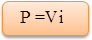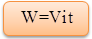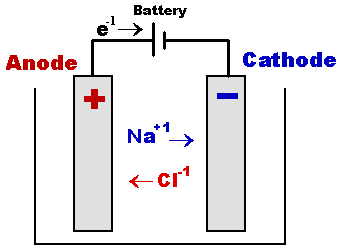Click to Chat

1800-1023-196

+91-120-4616500

CART 0

• 0

MY CART (5)

Use Coupon: CART20 and get 20% off on all online Study Material

ITEM
DETAILS
MRP
DISCOUNT
FINAL PRICE
Total Price: Rs.

There are no items in this cart.
Continue Shopping• Complete Physics Course - Class 11
• OFFERED PRICE: Rs. 2,968
• View Details

Thermal and Chemical Effect of current

Table of Content

Chemical Energy

Electrical Energy

Emf

Heating effect of Electric Current (Joule’s Law)

Electric Power

Units of Power

Electric Energy

Units of Electric Energy

Applications of Heating Effect of Electric Current

Electrolysis

Problem (JEE Main)

Related Resources

The flow of electric current in a circuit is affected by a number of factors. We shall discuss in detail these effects but first let us have a glimpse of the terms used in this context:

Chemical Energy

The energy stored in bonds of chemical compounds is called the chemical reaction. This energy is generally released in a chemical reaction which also produces heat as a by-product. When the chemical energy is released from a substance, it gets transformed into an entirely new substance. Some examples of stored chemical energy include battery, biomass, petroleum, natural gas, coal etc.

Electrical Energy

The energy carried and transmitted by moving electrons in an electric conductor is called the electrical energy. This form of energy can be used and transmitted very easily but cannot be seen. The electricity generating plants do not create energy. They just transform the other form of energy into electricity. A battery transforms stored chemical energy in the form of electrons moving through the wire. Lightning is an apt example of electrical energy in nature.

Emf

Emf is basically an abbreviation of electromotive force. It refers to the voltage generated by a battery or by the magnetic force of Faraday’s Law according to which the time varying magnetic force will produce the electric current. Though it is called the electromotive force but it is not actually a force. It is in fact a potential or the energy per unit charge.

Heating effect of Electric Current (Joule’s Law)The heat which is produced due to the flow of current within an electric wire, is expressed in Joules. Now the mathematical representation or explanation of Joule’s law is given in the following manner.

(a) Law of current

The amount of heat produced in current conducting wire, is proportional to the square of the amount of current that is flowing through the circuit, when the electrical resistance of the wire and the time of current flow is constant.

i.e. H ∝ i2 (When R & t are constant)

This verifies the law of electric current

(b) Law of time (R and i kept constant)

Let ‘H1’ and ‘H2’ be the amounts of heat produced when a current ‘i’ flows through the same resistance R for times t1 and t2 respectively. Experimentally, it is observed that,

H1/H2 = t1/t2

indicating that H ∝ t.

This verifies the law of time.

(c) Law of resistance (i and t kept constant)

Let ‘H1’ be the amount of heat produced when an electric current ‘i’ flows through a wire of resistance ‘R1’ for a time ‘t’. Replace the wire by another wire of resistance ‘R2’. Let ‘H2’ be the heat produced when same current passes through it for same time.

Experimentally, it is observed that, H1/H2 = R1/R2

indicating that H ∝ R.

This verifies the law of resistance.

While stating Joule’s law, we should be clear about the quantity which is maintained constant.

(i) When current is kept constant,

H ∝ i2

H ∝ R

H ∝ t

(ii) When potential difference is kept constant,

H ∝ V2

H ∝ 1/R

H ∝ t

Electric PowerWhen a current ‘i’ flows through a conductor for a time ‘t’, work done ‘W’ is given by

W = Vit

Here, ‘V’ is the potential difference across the two ends of the conductor.

Since power is defined as the rate of doing work,

Power, P = work/time = Vit/t

Or,Thus, electric power is the product of electric current and the potential difference due to which the current flows.

Units of Power

Power is measured in ‘Watt’.

1 watt = 1 volt  1 amp

Power of an electric circuit is said to be 1 watt if a current of 1 amp flows through it when there is a potential difference of 1 volt across its ends.

A bigger unit of power called ‘Kilowatt’ is also commonly used.

1 kilowatt = 1000 watt

Electric Energy

Electric energy is defined as the amount of work done in sending a current through a conductor. If ‘i’ is the current flowing through the conductor for a time ‘t’ when ‘V’ is the potential difference across the two ends of the conductor, work done W is given by,This is also known as Electric Energy.

Units of Electric Energy

If ‘V’ is taken in volt ‘i’ in amp andf ‘t’ in second, ‘W’ is given in joule.

1 joule = 1 volt  1 amp  1 sec = 1 watt  1 sec = 1 watt sec

A bigger unit of energy is ‘watt hour’.

1 watt hour = 1 watt  1 hour = 1 watt  3600 second

= 3600 watt second = 3600 joule = 3600  107 erg

In practice, a still bigger unit, kilowatt hour, is used.

1 kilowatt hour = 1000 watt hour

This is the unit which is used for calculations of electricity for domestic consumption and is called B.O.T (Board of Trade) Unit.

Applications of Heating Effect of Electric Current

The heating effect of electric current has many practical applications.  It is used in many domestic appliances such as an electric heater, an electric iron, a geyser, electric oven etc.

(a) An electric bulb contains a thin filament of metal like tungsten.  It has high melting point.  When current passes through the filament it is heated to high temperature and emits light.  This is the principle on which electric incandescent light bulb works.

(b) An electric iron used for ironing clothes consists of a coil of high resistance covered by insulating mica sheets and kept inside heavy metal block.  When electric current passes through the coil it gets heated.  The iron metal block gets heated and can be used for ironing clothes.

Refer this video to know more about on Joule’s law

ElectrolysisBehavior of substances to the passage of electric current through them can be classified into two categories:

(a) Substances which do not decompose due to the passage of electric current.

Example: Pure metals whether they are in solid or liquid form

(b) Substances which decompose due to the passage of electricity.

Example: Solution of copper sulphate, sodium chloride, etc. Such substances are called electrolytes.

The process of decomposition of electrolytes by the passage of electric current through them is called electrolysis.

The process of electrolysis is carried in a vessel called voltmeter. The electrolyte is put into the voltmeter. A source of e.m.f is connected across two conducting plates dipping into electrolyte.

The plates are called electrodes. The plate A connected with positive terminal of the source is called anode whereas the plate ‘C’ connected with negative terminal is called cathode.

Faraday gave the following two laws:

(a) The mass of ion deposited of an electrode in the process of electrolysis, is proportional to the quantity of charge that has passed through the electrolyte.

If ‘m’ is the mass of ion deposited on an electrode due to passage of charge ‘q’, then,

m ∝ q

But q = it

Here, ‘i’ is the current which flows for a time t.

So, m ∝ it

Or, m = zit     ….... (1)

Here ‘z’ is the constant of proportionality and is known as electro-chemical equivalent (e.c.e.) of the substance.

If i = 1 A, t = 1 s, m = z.

Therefore, electro – chemical equivalent of a substance is numerically equal to the mass of ion deposited when a charge of 1 C (or a current of 1 A passes through it for 1 s) passes through it.

e.c.e. varies from one substance to the other. Its units are gC-1.

(b) When same current passes through several electrolytes for the same time, the masses of various ions deposited at each of the electrodes are proportional to their chemical equivalents (equivalent weights).

If certain current passes through two electrolytes A and B for the same time,

weight of A deposited / weight of B deposited = chemical equivalent of A / chemical equivalent of B

It may be noted that chemical equivalent of an element is defined as the ratio of its atomic weight to its valence.

Chemical equivalent = atomic weight/valence

Let same charge ‘q’ be passed through two electrolytes having e.c.e.’s z1 and z2. Let m1 and m2 be the masses of ions liberated.

m1 = z1q

m2 = z2q  or m1/m2 = z1/z2       …... (2)

According to Faraday’s second law, m1/m2 = E1/E2        …... (3)

Here, ‘E1’ and ‘E2’ are the chemical equivalents of two electrolytes.

From equations (2) and (3), we get,

E1/E2 = z1/z2 or E1/z1 = E2/z2

That is, E/z = constant = F (say)                      …... (4)

‘F’ is known as a ‘faraday’.

from equation (1), z = m/it = m/q

Substituting in (4), we get,

F = E / (m/q) = Eq/m

If m = E, F = q

Thus, a faraday is the amount of charge required to liberate one gram equivalent of a substance during electrolysis.

For copper E = 31.5 g

z = 0.000329 gC-1

Substituting in equation (4), we get,

F = 31.5 g / 0.000329 gC-1 = 96500 C

Thus, a faraday represents a charge 96500 C.When electric energy is supplied to an electric bulb, the filament gets heated because of which it gives light. The heating of electric bulb happens because of heating effect of electric current.

When electric current is supplied to a purely resistive conductor, the energy of electric current is dissipated entirely in the form of heat and as a result, resistor gets heated. The heating of resistor because of dissipation of electrical energy is commonly known as Heating Effect of Electric Current.

Greater the current, greater will be the rate of collision and this results greater will be the heat produced.

?Faraday’s Laws of Electrolysis give the relationship between the amount of material liberated at the electrode and the amount of electric energy that is passed through the electrolyte.

Electrochemical equivalent of a substance is the mass of the substance liberated or deposited in electrolysis by the passage of 1 coulomb of charge.

Problem (JEE Main)

Power generated across a uniform wire connected across a supply is H. If the wire is cut into n equal parts and all the parts are connected in parallel across the same supply, the total power generated in the wire is

(a) H/n2

(b) n2H

(c) nH

(d) H/n

Solution

R' = R/n2

P = V2/R

Or P ∝ 1/R      If V is same

So, P'/P = R/R' = n2

Or, P' = n2P = n2H

Thus, from the above observation we conclude that, option (b) is correct.

Problem:

An electric bulb rates 220 V and 60 W is connected in series with another electric bulb rated 220 V and 40 W. The combination is connected across a 220 volt source of emf. Which bulb will glow brighter?

Solution:

We are given electric bulbs written in series.

R = V2 / P

Resistance of first bulb is R1 = V2 / P2

and resistance of the second bulb is R2 = V2 / P2

In series, same current will pass through each bulb.

Power developed across first bulb is P'1 = l2 V2 / P1,

and power developed across second bulb is P'2 = l2 V2 / P2 Þ P'1 / P'2 = P2 / P1

As P2 < P1, hence P2 / P1 < 1

This implies P'1 / P'2 < 1

So P'1 < P'2

The bulb rates 220 V & 40 W will glow brighter.Question 1

According to Joule’s law of heating, heat produced in a resistor is

(a) directly proportional to the square of current,

(b) inversely proportional to resistance

(c) directly proportional to the square of the time for which the current flows through

(d) directly proportional to the square of current,

Question 2

The S.I. unit of a power is,

(a) Henry

(b) Coulomb

(c) watt

(d) watt - hour

Question 3

The filament of an electric bulb is made of

(a) carbon

(b) aluminum

(c) tungsten

(d) nickel

Question 4

The rating of a fuse wire is always expressed in

(a) ampere-hours

(b) ampere-volts

(c) kWh

(d) amperesQ.1
Q.2
Q.3
Q.4

a

c

c

d

Related Resources

You might like to Electric Current and Curent Density.

For getting an idea of the type of questions asked, refer the  Previous Year Question Papers.

To read more, Buy study materials of Current Electricity comprising study notes, revision notes, video lectures, previous year solved questions etc. Also browse for more study materials on Physics here.### Course Features

• 101 Video Lectures
• Revision Notes
• Previous Year Papers
• Mind Map
• Study Planner
• NCERT Solutions
• Discussion Forum
• Test paper with Video Solution